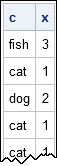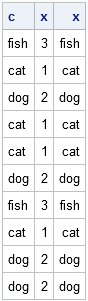The other day someone posted the following question to the SAS-L discussion list:

Is there a SAS PROC out there that takes a multi-category discrete variable with character categories and converts it to a single numeric coded variable (not a set of dummy variables) with the character categories assigned as formats?

The "Macro Maven," Ron Fehd, responded with a link to a SAS macro that uses the results of PROC FREQ to perform this task. (For details and related macros, see Ron's 2008 SAS Global Forum paper.)

I'm not much of a macro maven myself, but I am a SAS/IML maven. This post presents a SAS/IML program that performs the recoding task. To begin, suppose we have some character data:

```data MyData; input pets : \$4. @@; datalines; fish cat dog cat cat dog fish cat dog dog ;```

The following SAS/IML statements read the PETS variable from the data and use the UNIQUE function to create a numeric vector that contains the values 1 through k, where k is the number of unique values of the character variable:

```proc iml; use MyData; read all var {pets} into c; close MyData;   u = unique(c); /* get unique (sorted) values */ x = j(nrow(c), 1); /* allocate numerical vector */ do i = 1 to ncol(u); /* for each unique value */ idx = loc(c=u[i]); /* find obs with that value */ x[idx] = i; /* assign i to those obs */ end; print c x;```I've written about this UNIQUE-LOC technique in my book and in previous blog posts. It is an efficient way to manipulate categorical variables in the SAS/IML language. In this example, it is used to recode the character values into numeric values.

The second part of this task is to create a user-defined format to map the numeric values back to their corresponding character values. To do this, you can take advantage of a feature in SAS/IML 9.22 and beyond: the ability to call SAS procedures from within your SAS/IML program.

For the current data, a PROC FORMAT call that assigns the values 1–3 to the strings "cat," "dog," and "fish" would look like the following:

```/* we want to submit this code to SAS */ proc format; value PETS 1='cat' 2='dog' 3='fish'; run;```

Notice that the values on the left side of the equal signs are the elements of the SAS/IML vector 1:ncol(u) whereas the values on the right side of the equal signs are the elements of u. Consequently, you can use string concatenation to create a string of number-character pairs. If we can pass this string from the SAS/IML language into PROC FORMAT, we can solve the second task.

By using the SUBMIT and ENDSUBMIT statements, you can not only call SAS procedures, but you can also pass information from a SAS/IML vector (or matrix) into the procedure. All you need to do is place the name of the SAS/IML vector on the SUBMIT statement. You can reference the contents of that vector within the submit block by preceding the vector's name with an ampersand, as follows:

```ValuePairs = char(1:ncol(u)) + "='" + u + "'"; /* string concatenation */ submit ValuePairs; proc format; value PETS &ValuePairs; /* resolves to contents of the vector */ run; endsubmit;   print c x x[format=PETS.];```In the output table, the first column is the original data, the second column is the recoded numeric data, and the third column is the result of applying the new PETS. format to the numeric data.

Notice that SUBMIT/ENDSUBMIT block looks like it substituting the value of macro into the PROC FORMAT call, but it is not. There is no macro variable called ValuePairs. Instead, the ampersand means "insert the contents of the SAS/IML vector before submitting the code to SAS."

It is trivial to turn this program into a real macro that can be called on an arbitrary variable in an arbitrary data set to create a format with a given name.

ShareDistinguished Researcher in Computational Statistics

Rick Wicklin, PhD, is a distinguished researcher in computational statistics at SAS and is a principal developer of SAS/IML software. His areas of expertise include computational statistics, simulation, statistical graphics, and modern methods in statistical data analysis. Rick is author of the books Statistical Programming with SAS/IML Software and Simulating Data with SAS.

1.2.•